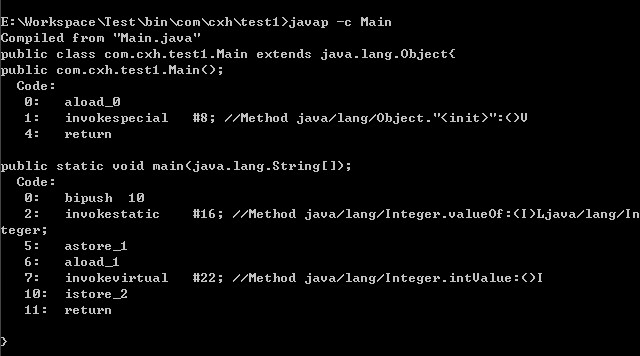自动拆装箱

int（4字节） Integer
byte（1字节） Byte
short（2字节） Short
long（8字节） Long
float（4字节） Float
double（8字节） Double
char（2字节） Character
boolean（未定） Boolean

装箱和拆箱是如何实现的

public class Main {
public static void main(String[] args) {

Integer i = 10;
int n = i;
}
}Java Integer的缓存策略

package com.javapapers.java;

public class JavaIntegerCache {
public static void main(String... strings) {

Integer integer1 = 3;
Integer integer2 = 3;

if (integer1 == integer2)
System.out.println("integer1 == integer2");
else
System.out.println("integer1 != integer2");

Integer integer3 = 300;
Integer integer4 = 300;

if (integer3 == integer4)
System.out.println("integer3 == integer4");
else
System.out.println("integer3 != integer4");

}
}

integer1 == integer2
integer3 != integer4

Java 中 Integer 缓存实现

• 上面的规则适用于整数区间 -128 到 +127。

• 这种 Integer 缓存策略仅在自动装箱（autoboxing）的时候有用，使用构造器创建的 Integer 对象不能被缓存。

Java 编译器把原始类型自动转换为封装类的过程称为自动装箱（autoboxing），这相当于调用 valueOf 方法

Integer a = 10; //this is autoboxing
Integer b = Integer.valueOf(10); //under the hood

/**
* Returns an {@code Integer} instance representing the specified
* {@code int} value.  If a new {@code Integer} instance is not
* required, this method should generally be used in preference to
* the constructor {@link #Integer(int)}, as this method is likely
* to yield significantly better space and time performance by
* caching frequently requested values.
*
* This method will always cache values in the range -128 to 127,
* inclusive, and may cache other values outside of this range.
*
* @param  i an {@code int} value.
* @return an {@code Integer} instance representing {@code i}.
* @since  1.5
*/
public static Integer valueOf(int i) {
if (i >= IntegerCache.low && i <= IntegerCache.high)
return IntegerCache.cache[i + (-IntegerCache.low)];
return new Integer(i);
}

IntegerCache 类

IntegerCache 是 Integer 类中一个私有的静态类。我们来看看这个类，有比较详细的文档，可以提供我们很多信息。

/**
* Cache to support the object identity semantics of autoboxing for values between
* -128 and 127 (inclusive) as required by JLS.
*
* The cache is initialized on first usage.  The size of the cache
* may be controlled by the {@code -XX:AutoBoxCacheMax=} option.
* During VM initialization, java.lang.Integer.IntegerCache.high property
* may be set and saved in the private system properties in the
* sun.misc.VM class.
*/

private static class IntegerCache {
static final int low = -128;
static final int high;
static final Integer cache[];

static {
// high value may be configured by property
int h = 127;
String integerCacheHighPropValue =
sun.misc.VM.getSavedProperty("java.lang.Integer.IntegerCache.high");
if (integerCacheHighPropValue != null) {
try {
int i = parseInt(integerCacheHighPropValue);
i = Math.max(i, 127);
// Maximum array size is Integer.MAX_VALUE
h = Math.min(i, Integer.MAX_VALUE - (-low) -1);
} catch( NumberFormatException nfe) {
// If the property cannot be parsed into an int, ignore it.
}
}
high = h;

cache = new Integer[(high - low) + 1];
int j = low;
for(int k = 0; k < cache.length; k++)
cache[k] = new Integer(j++);

// range [-128, 127] must be interned (JLS7 5.1.7)
assert IntegerCache.high >= 127;
}

private IntegerCache() {}
}

Javadoc 详细的说明这个类是用来实现缓存支持，并支持 -128 到 127 之间的自动装箱过程。最大值 127 可以通过 JVM 的启动参数 -XX:AutoBoxCacheMax=size 修改。 缓存通过一个 for 循环实现。从小到大的创建尽可能多的整数并存储在一个名为 cache 的整数数组中。这个缓存会在 Integer 类第一次被使用的时候被初始化出来。以后，就可以使用缓存中包含的实例对象，而不是创建一个新的实例(在自动装箱的情况下)。

其他缓存的对象

• 有 ByteCache 用于缓存 Byte 对象
• 有 ShortCache 用于缓存 Short 对象
• 有 LongCache 用于缓存 Long 对象
• 有 CharacterCache 用于缓存 Character 对象

Byte，Short，Long 有固定范围: -128 到 127。对于 Character, 范围是 0 到 127。除了 Integer 可以通过参数改变范围外，其它的都不行。